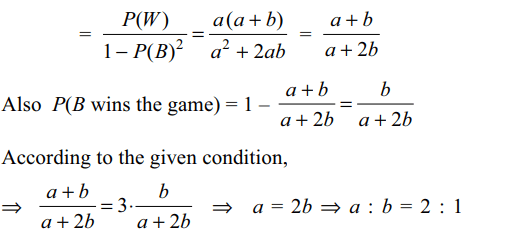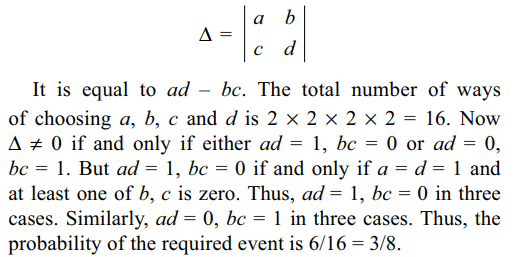## Probability Questions and Answers Part-1

1. A fair coin is tossed 2019 times. Probability of getting at least 1010 heads is
a) $\frac{1}{2}$
b) $\frac{1}{4}$
c) $\frac{1}{2^{1009}}$
d) $\frac{1}{2^{1010}}$

Explanation: Let n = 1009, then 2n + 1 = 20192. From the first 3n(n ≥ 2) natural numbers two numbers are picked up at random without replacement. Let P denote the probability that the absolute value of the difference between the numbers is less than n, then P is equal to
a) $\frac{2\left(n-1\right)}{3n-1}$
b) $\frac{5\left(n-1\right)}{3\left(3n-1\right)}$
c) $\frac{n-1}{3n-1}$
d) $\frac{n+1}{3\left(3n-1\right)}$

Explanation: Two numbers a, b from first 3n natural numbers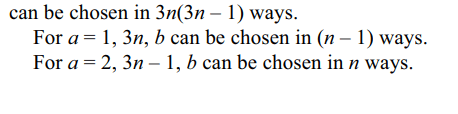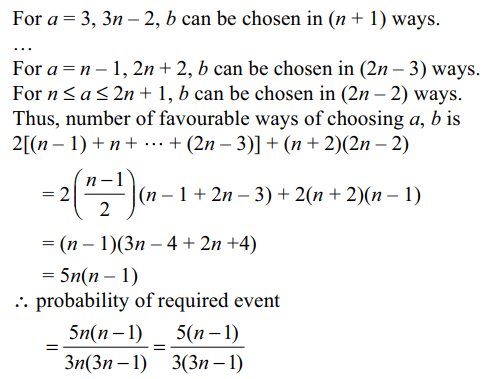3. A box conatins 2n cards numbered $2^{0},2^{1},2^{2},....,2^{2n-1}$     . Two cards are taken out at random from the box, at random without replacement. Probability that sum of the numbers on the card is divisible by 3 is
a) $\frac{n}{2n-1}$
b) $\frac{n-1}{2n-1}$
c) $\frac{n+1}{2n}$
d) $\frac{1}{2}$

Explanation: Let the smaller card bear number a and the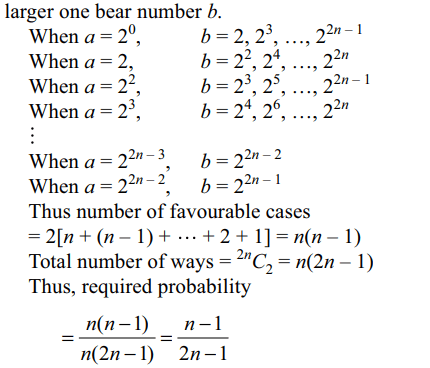4. An unbiased cubical die is thrown n times. If $x_{i}$= outcome on the ith throw and$X=gcd \left(x_{1},x_{2},...., x_{n}\right)$      , then E(X) is equal to
a) $\frac{1}{6^{n}}\left(6^{n}+3^{n}+2^{n}\right)$
b) $\frac{1}{6^{n}}\left(6^{n}+3^{n}+2^{n}+1\right)$
c) $\frac{1}{6^{n}}\left(6^{n}+3^{n}+2^{n+1}+8\right)$
d) $\frac{1}{6^{n}}\left(6^{n}+3^{n}+2^{n+2}+8\right)$

Explanation: Sample space of the random experiment is5. There are 8 blue and 2 red balls in a bag. Each time one ball is drawn and replaced by a blue one. The probability of drawing the last red ball on the fourth draw is
a) 0.0434
b) 0.0438
c) 0.0444
d) 0.0453

Explanation: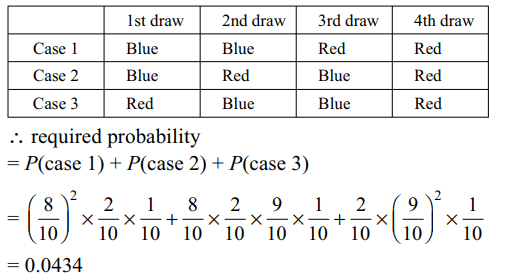6. There are m persons sitting in a row. Two of them are selected at random. The probability that the two selected persons are not together is
a) 2/m
b) 1/m
c) $\frac{m\left(m-1\right)}{\left(m+1\right)\left(m+2\right)}$
d) $1-\frac{2}{m}$

Explanation: The total number of ways of selecting two persons out of m is7. Three six-faced fair dice are thrown together. The probability that the sum of the numbers appearing on the dice is $k\left(3\leq k\leq8\right)$   is
a) $\frac{\left(k-1\right)\left(k-2\right)}{432}$
b) $\frac{k\left(k-1\right)}{432}$
c) $\frac{k^{2}}{432}$
d) $k^{2}/216$

Explanation: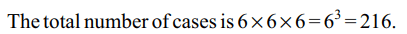8. Two squares are chosen at random on a chessboard. The probability that they have a side in common is
a) 1/9
b) 2/7
c) 1/18
d) 2/9

Explanation: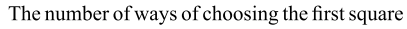9. A bag contains a white and b black balls. Two players, A and B alternately draw a ball from the bag, replacing the ball each time after the draw till one of them draws a white ball and wins the game. A begins the game. If the probability of A winning the game is three times that of B, the ratio a : b is
a) 1 : 1
b) 1 : 2
c) 2 : 1
d) 1 : 3